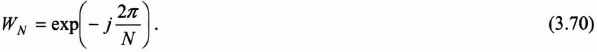## 3.4. The fast Fourier transform (FFT)

In 1965, Cooley and Tuekey proposed a fast algorithm for calculating the discrete Fourier transform. With real signals, the direct calculation of equation (3.66) requires 2N2 multiplications and 2N(N − 1) additions. With complex signals, the computational cost reaches 4N2 multiplications and 2N(2N − 1) additions. The fast method consists of operating by dichotomy, which reduces, as we will see later, to a calculatory complexity as N log2 (N).

From now on, to simplify presentation, we use the example of a fast Fourier transform on N = 8 points.We introduce the coefficient WN called the “Twiddle factor”, which corresponds to the complex root of the unity represented by:We can then rewrite equation (3.69) in the form:For N = 8, equation (3.71) leads to the following matricial equation:The complex roots of the unity have specific qualities that can be exploited to simplify equation (3.72). Actually, the “Twiddle factors” satisfy the following properties and . We thus show the redundancy of the WN coefficients. This is a reduction of this redundancy ...

Get Digital Filters Design for Signal and Image Processing now with the O’Reilly learning platform.

O’Reilly members experience books, live events, courses curated by job role, and more from O’Reilly and nearly 200 top publishers.